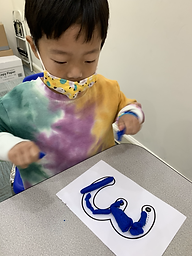Ms. Kayla

Target 1​

Lesson Type:

Continuation

Geometry

:

Shape

Identify and compare basic geometric shapes, regardless of orientation or size.

1:

Identify simple geometric shapes in a variety of colors, sizes, and orientations.

2:

Independently identify squares, rectangles, circles, and triangles.

3:

Identify basic geometric shapes that best represent real-world objects.

Pre-K

Vocabulary:

Shape, Circle, Rectangle, Triangle, Oval, Square

Activities:

1. Students picked an object to construct using geometric shapes.

2. Students identified the shapes needed to construct an image.Home Exploration

Guiding Questions:Absent Students:

Target 2

:

1:

Write numbers correctly.

2:

Recognize a number without having to see its position in the count sequence.

Pre-K

Vocabulary:

Number, Zero, One, Two, Three, Four, Five, Six, Seven, Eight, Nine, Trace, Write

Activities:

1. Students were randomly given a number to identify.

2. Students first traced the number with their finger and then with a marker.

3. Students also used playdough to represent the given digit.Home Exploration

Guiding Questions:Target 3

:

1:

Compare objects by size, length, weight, and capacity.

Pre-K

Vocabulary:

Sort, Numbers, Graph, One, Two, Three, Four, Five, Six, Seven, Eight, Nine, Ten, Eleven, Twelve

Activities:

1. Students sorted farm animals (horses, sheep, pigs, chickens, dogs, cats, cows, etc.).

2. Students counted the number of animals in each group with help.

3. Students discussed which group of farm animals had the most.

4. Students lined up the animals in rows to represent a picture graph/pictograph (sort of).

5. Students also looked at the number of letters in their names.Home Exploration